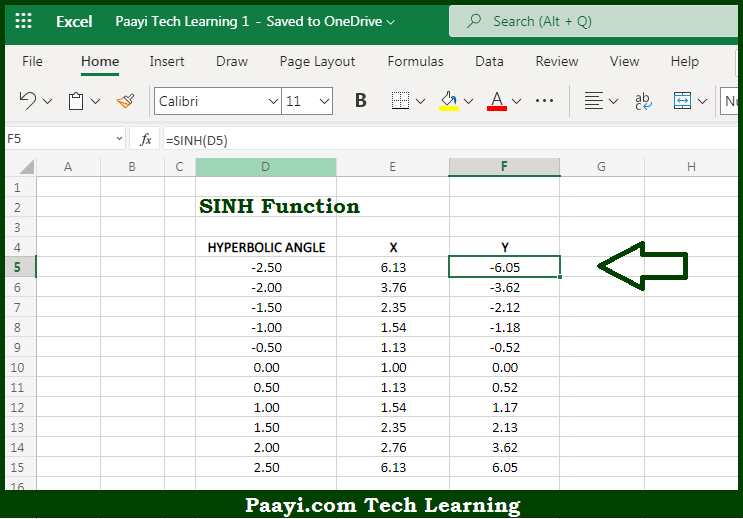# Learn How to Use Microsoft Excel SINH Function

Written by | 0 Comments | 530 Views

In this article, you will learn how to use the Microsoft Excel SINH function and its prime function in Microsoft Excel. You will also get to know the Microsoft Excel SINH function return value and syntax with the help of some examples.

Microsoft Excel SINH Function

The main purpose of the Microsoft Excel SINH function is to get the hyperbolic sine of the number. That implies, with the help of SINH function you can able to return the hyperbolic sine of the number. In geometry, the SINH function returns the y-component of the point on the unit hyperbola defined by a hyperbolic angle. So, with the help of SINH function, you can able to get the sine of an angle provided in the radians.

Return Value of SINH Function

The return value will be the hyperbolic sine value.

Syntax of SINH Function

=SINH(number)

Where the arguments:

• number: This is the hyperbolic angle.

## How to Use Microsoft Excel SINH Function?So we know that Microsoft Excel SINH function you can able to get the hyperbolic sine of the number. That implies, with the help of the SINH function you can able to return the hyperbolic sine of the number. In geometry, the SINH function returns the y-component of the point on the unit hyperbola defined by a hyperbolic angle. So, with the help of the SINH function, you can able to get the sine of an angle provided in the radians.